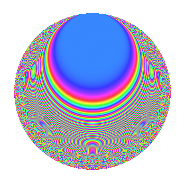# Properties

 Label 1503.2.cLevel 1503 Weight 2 Character orbit c Rep. character $$\chi_{1503}(1502,\cdot)$$ Character field $$\Q$$ Dimension 56 Newform subspaces 1 Sturm bound 336 Trace bound 0

# Related objects

## Defining parameters

 Level: $$N$$ $$=$$ $$1503 = 3^{2} \cdot 167$$ Weight: $$k$$ $$=$$ $$2$$ Character orbit: $$[\chi]$$ $$=$$ 1503.c (of order $$2$$ and degree $$1$$) Character conductor: $$\operatorname{cond}(\chi)$$ $$=$$ $$501$$ Character field: $$\Q$$ Newform subspaces: $$1$$ Sturm bound: $$336$$ Trace bound: $$0$$

## Dimensions

The following table gives the dimensions of various subspaces of $$M_{2}(1503, [\chi])$$.

Total New Old
Modular forms 172 56 116
Cusp forms 164 56 108
Eisenstein series 8 0 8

## Trace form

 $$56q - 48q^{4} + O(q^{10})$$ $$56q - 48q^{4} + 32q^{16} - 8q^{19} + 16q^{22} + 64q^{25} - 32q^{28} + 40q^{31} + 56q^{49} - 32q^{58} + 24q^{61} - 56q^{76} + 72q^{88} - 56q^{94} - 48q^{97} + O(q^{100})$$

## Decomposition of $$S_{2}^{\mathrm{new}}(1503, [\chi])$$ into newform subspaces

Label Dim. $$A$$ Field CM Traces $q$-expansion
$$a_2$$ $$a_3$$ $$a_5$$ $$a_7$$
1503.2.c.a $$56$$ $$12.002$$ None $$0$$ $$0$$ $$0$$ $$0$$

## Decomposition of $$S_{2}^{\mathrm{old}}(1503, [\chi])$$ into lower level spaces

$$S_{2}^{\mathrm{old}}(1503, [\chi]) \cong$$ $$S_{2}^{\mathrm{new}}(501, [\chi])$$$$^{\oplus 2}$$

## Hecke characteristic polynomials

There are no characteristic polynomials of Hecke operators in the database